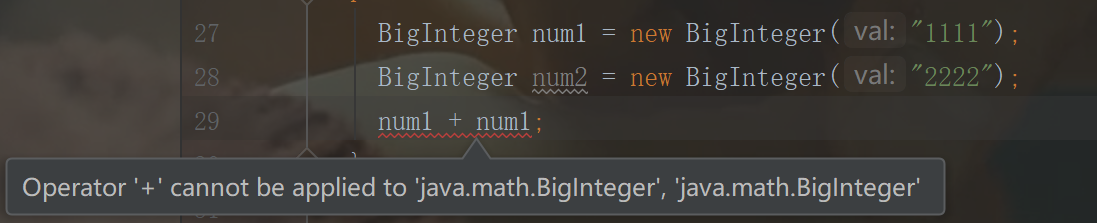# Java工具类——数学相关的类

#### 一、数学类介绍

Math类 java.lang包，不需要导包 默认继承Object基类
BigInteger类 java.math包，需要导包 继承自Number类，实现了Serializable, Comparable接口
BigDecimal类 java.math包，需要导包 继承自Number类，实现了Serializable, Comparable接口

#### 二、Math类

``````public final class Math {}
``````

#### 1、Math类构造方法

``````/**
* Don't let anyone instantiate this class.
*/
private Math() {}
``````

#### 2、Math类常用的方法

• abs（）方法返回给定数的绝对值，方法提供了4个不同参数类型重载方法（int, long, float, double）
``````int abs1 = Math.abs(-1);
long abs2 = Math.abs(-3l);
float abs3 = Math.abs(-1.2f);
double abs4 = Math.abs(-3.923);
System.out.println(abs1);
System.out.println(abs2);
System.out.println(abs3);
System.out.println(abs4);
``````
• ceil（）方法返回大于或等于参数且等于一个数学整数的最小的双精度值，可以理解为向上取整。
``````System.out.println(Math.ceil(-1.3));//-1.0
System.out.println(Math.ceil(1.9));//2.0
System.out.println(Math.ceil(-7.9));//-7.0
System.out.println(Math.ceil(123));//123.0
``````
• floor（）方法返回最大的双精度值，该双精度值小于或等于参数，并且等于一个数学整数，可以理解为向下取整。
``````System.out.println(Math.floor(-1.3));//-2.0
System.out.println(Math.floor(1.9));//1.0
System.out.println(Math.floor(1.3));//1.0
System.out.println(Math.floor(-7.9));//-8.0
System.out.println(Math.floor(123));//123.0
``````
• round（）方法返回与参数最接近的整型数，四舍五入为正无穷，其实就是四舍五入的整数。
``````System.out.println(Math.round(-1.3));//-1
System.out.println(Math.round(1.9));//2
System.out.println(Math.round(1.3));//1
System.out.println(Math.round(-7.9));//-8
System.out.println(Math.round(123));//123
``````
• max（）方法返回最大值，该方法提供了4个不同参数类型重载方法（int,long,float,double）
``````System.out.println(Math.max(1, 3));//3
System.out.println(Math.max(-4, -5));//-4
System.out.println(Math.max(1.8, 1.92));//1.92
System.out.println(Math.max(-4f, -4f));//-4.0
``````
• min（a, b）方法返回最小值，该方法提供了4个不同参数类型重载方法（int, long, float, double）
``````System.out.println(Math.min(1, 3));//1
System.out.println(Math.min(-4, -5));//-5
System.out.println(Math.min(1.8, 1.92));//1.8
System.out.println(Math.min(-4f, -4f));//-4.0
``````
• pow（a, b）方法返回 a 的 b 次方，其中参数和返回值都是 double 类型的
``````System.out.println(Math.pow(3, 3));//27.0
System.out.println(Math.pow(3.2, 5));//335.5443200000001
``````
• random（）方法生成一个 double 类型的随机数，范围是[ 0.0, 1.0)，注意是左闭右开。
``````System.out.println(Math.random());//0.4128879706448445
System.out.println(Math.random());//0.9024029619163387
System.out.println(Math.random());//0.4265563513755902
``````

#### 三、BigInteger类

BigInteger 顾名思义，其实就是叫大整数，也就是说只能存储整型的数，我们通过构造方法和常用方法来了解BigInteger 类。

#### 1、构造方法

BigInteger 类有8个构造方法，其中有2个私有构造方法。8个构造方法分别是：

``````private BigInteger(int[] val){}
``````
``````private BigInteger(int signum, int[] magnitude){}
``````
``````public BigInteger(byte[] val){}
``````
``````public BigInteger(String val){}
``````
``````public BigInteger(String val, int radix) {}
``````
``````public BigInteger(int signum, byte[] magnitude){}
``````
``````public BigInteger(int numBits, Random rnd){}
``````
``````public BigInteger(int bitLength, int certainty, Random rnd){}
``````

#### 2、类中常用的方法`````` private static void test6() {
BigInteger num1 = new BigInteger("1111");
BigInteger num2 = new BigInteger("2222");
//加法
//减法
BigInteger subtract = num2.subtract(num1);
//乘法
final BigInteger multiply = num1.multiply(num2);
//除法（取整）
BigInteger divide = num2.divide(num1);
//除法（取余）
BigInteger mod = num2.mod(num1);
System.out.println(subtract);//1111
System.out.println(multiply);//2468642
System.out.println(divide);//2
System.out.println(mod);//0
}
``````

#### 1、构造方法

BigDecimal 类的构造方法有很多，最常用的是以下四个：

``````public BigDecimal(double val) {
``````
``````public BigDecimal(int val) {}
``````
``````public BigDecimal(String val) {}
``````
``````public BigDecimal(long val) {}
``````

#### 2、类中常用的方法

``````private static void test7() {
BigDecimal num1 = new BigDecimal("1.23");
BigDecimal num2 = new BigDecimal("2.46");
//加法
//减法
BigDecimal subtract = num2.subtract(num1);
//乘法
final BigDecimal multiply = num1.multiply(num2);
//除法（取整）
BigDecimal divide = num2.divide(num1);

System.out.println(subtract);//1.23
System.out.println(multiply);//3.0258
System.out.println(divide);//2
}
``````

``````public BigDecimal setScale(int newScale)
``````
``````public BigDecimal setScale(int newScale, int roundingMode)
``````
``````public BigDecimal setScale(int newScale, RoundingMode roundingMode)
``````

``````
public final static int ROUND_UP =           0;

public final static int ROUND_DOWN =         1;

public final static int ROUND_CEILING =      2;

public final static int ROUND_FLOOR =        3;

public final static int ROUND_HALF_UP =      4;

public final static int ROUND_HALF_DOWN =    5;

public final static int ROUND_HALF_EVEN =    6;

public final static int ROUND_UNNECESSARY =  7;
``````

• ROUND_UP 模式

UP的意思就是向上的意思，可以理解为加的意思。比如我保留了三位小数，那么我不管你后面的小数值如何（除了0），我都给你加一，就相当于四舍五入的五入。比如下面这个代码，我保留3为小数，模式设置为ROUND_UP模式，那么如果最后一位是0，那么结果就是9.461，否则就是9.462

``````BigDecimal bigDecimal = new BigDecimal("9.4610");
BigDecimal result = bigDecimal.setScale(3, BigDecimal.ROUND_UP);
``````
• ROUND_DOWN 模式

``````BigDecimal bigDecimal = new BigDecimal("9.4611");
BigDecimal result = bigDecimal.setScale(3, BigDecimal.ROUND_DOWN);
``````
• ROUND_CEILING 模式

• ROUND_FLOOR 模式

#### 五、小结